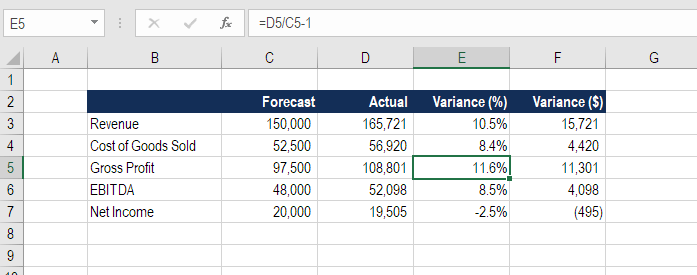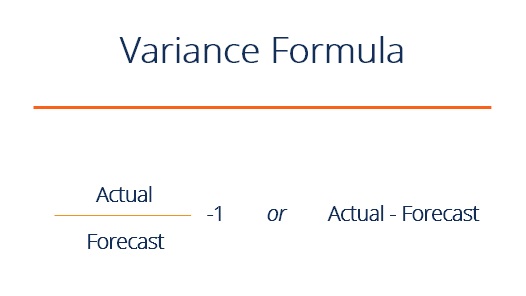# Variance Formula

Calculating the difference between a forecast and the actual result

## How to Calculate Variance?

The variance formula is used to calculate the difference between a forecast and the actual result. The variance can be expressed as a percentage or an integer (dollar value or the number of units).  Variance analysis and the variance formula play an important role in corporate financial planning and analysis (FP&A) to help evaluate results and make informed decisions for the business going forward.### What is the Variance Formula?

There are two formulas to calculate variance:

Variance % = Actual / Forecast – 1

Or

Variance \$ = Actual – ForecastIn the following paragraphs, we will break down each of the formulas in more detail.

### Percent variance formula

As the name implies, the percent variance formula calculates the percentage difference between a forecast and an actual result.

In the example analysis above we see that the revenue forecast was \$150,000 and the actual result was \$165,721.  Therefore, we take \$165,721 divided by \$150,000 less one and express that number as a percentage, which is 10.5%.

This is an example of an outperformance, a positive variance or a favorable variance.

### Dollar variance formula

The formula for dollar variance is even simpler, it’s equal to the actual result subtracted from the forecast number.  If the units are dollars, this gives us the dollar variance.  This formula can also work for the number of units or any other type of integer.

In the same example as above, revenue forecast was \$150,000 and the actual result was \$165,721. We now take \$165,721 and subtract \$150,000 to get a variance of \$15,721.### Favorable vs unfavorable variance

Since variance analysis is performed on both revenues and expenses, it’s important to carefully distinguish between a positive or negative impact.  For this reason, instead of saying positive, negative, over or under, the terms favorable and unfavorable are used, as they clearly make the point.

For example, if a cost has a negative difference to the forecast (lower than expected), that’s a favorable variance since it’s better to have costs lower rather than higher.

### Variance in budgeting and forecasting

The variance formula is useful in budgeting and forecasting when analyzing results.  The job of a financial analyst is to measure results, compare them to the budget/forecast, and explain what caused any difference.

In the Financial Planning & Analysis department at a company, the role of FP&A is to present management with accurate, timely, and insightful information so they can make effective decisions about the business going forward.

Using the variance formula and presenting this type of information is critical in FP&A.

• Marginal cost formula
• Analysis of financial statements
• Waterfall chart template
• Types of graphs in Excel

### Financial Analyst Training

Get world-class financial training with CFI’s online certified financial analyst training program!

Gain the confidence you need to move up the ladder in a high powered corporate finance career path.

Learn financial modeling and valuation in Excel the easy way, with step-by-step training.